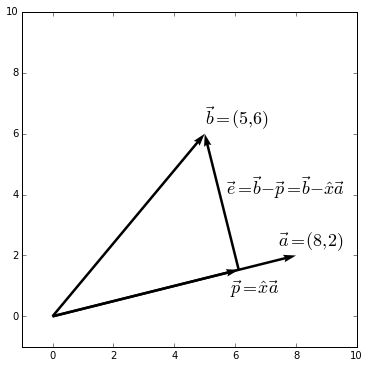As a simple example we project on to a line. First, calculate $e$ and $p$.

\begin{align} 0 &= \vec{e} \bullet{}\vec{a} && \vec{e} \perp \vec{a} \text{ so the dot product is zero}\\ 0 &= (\vec{b}-\hat{x}\vec{a})\bullet{}\vec{a} && \text{notice } \vec{e} = \vec{b}-\vec{p} = \vec{b}-\hat{x}\vec{a}\\ 0 &= \vec{b}\bullet \vec{a} - \vec{a} \hat{x}\bullet \vec{a} && \text{distribute } \vec{a}\\ 0 &= (\vec{b} \bullet \vec{a}) - \hat{x} (\vec{a} \bullet \vec{a}) && \text{notice } \hat{x} \text{ is a scalar, so the last term can be } \hat{x} \vec{a} \bullet \vec{a}\\ \hat{x} (\vec{a} \bullet \vec{a}) &= (\vec{b} \bullet \vec{a})\\ \hat{x} &= \dfrac{\vec{b} \bullet \vec{a}}{\vec{a} \bullet \vec{a}} \implies \vec{p} = \dfrac{\vec{b} \bullet \vec{a}}{\vec{a} \bullet \vec{a}} \vec{a} && \text{because } \vec{p} = \hat{x}\vec{a} \end{align}

We can summarize the projection to the matrix as, $\vec{p} = P\vec{b}$. This is easy to find if we present the equation the other way around. Notice that with vectors the order is inconsequential, because $\vec{a} \bullet \vec{b} = \vec{b} \bullet \vec{a}$

\begin{align} 0 &= \vec{a} \bullet \vec{e} && \vec{a}, \vec{e} \text{ on opposite sides}\\ 0 &= (\vec{a} \bullet \vec{b}) - \hat{x}(\vec{a} \bullet \vec{a})\\ \hat{x}(\vec{a} \bullet \vec{a}) &= (\vec{a} \bullet \vec{b})\\ \hat{x} &= \dfrac{\vec{a} \bullet \vec{b}}{\vec{a} \bullet \vec{b}} = \dfrac{\vec{a}^T\vec{b}}{\vec{a}^T\vec{a}} \end{align}

With $\hat{x}$ in this form, we can calculate the permutation matrix, $P$ from $\vec{p} = P\vec{b}$

\begin{align} \vec{p} &= \vec{a}\dfrac{\vec{a}^T\vec{b}}{\vec{a}^T\vec{a}}\\ \vec{p} &= \dfrac{\vec{a}\vec{a}^T}{\vec{a}^T\vec{a}}\vec{b} \implies P = \dfrac{\vec{a}\vec{a}^T}{\vec{a}^T\vec{a}} && \text{because } \vec{p}= P\vec{b} \end{align}

## Project Onto a Plane in $\mathbb{R}^3$

Note that $\vec{a}^T\vec{a}$ in the denominator evaluates to a scalar. If we want to project onto a plane, the denominator becomes $A^TA$, which evaluates to a matrix.

• Division by a matrix has no meaning. You cannot divide by a martix.
• In arithmatic, we undo multiplication with division.
• To undo the effects of a matrix multiplication, we multiply the inverse of the matrix. $A^{-1}A\vec{b}=\vec{b}$
• We must re-arrange the equation so that we use inverse matrices instead of division.

We can think of projecting onto a plane as projecting onto multiple vectors. To project $\vec{b}$ onto $A$, we are looking for the vector $\hat{x}$, such that $\vec{p}=A\hat{x}$, where $\vec{p}$ is the point on the plane closest to $\vec{b}$. The first step is to find the vector $\hat{x}$.

Like the first example, we define the error vector, $\vec{e}$, as the vector that goes from the plane to $\vec{b}$

$\begin{equation*} \vec{e} = \vec{b} - A\hat{x} \end{equation*}$

Assume $A$ is a matrix made of two vectors, $a_1$ and $a_2$ in $\mathbb{R}^3$:

$\begin{equation} A = \begin{bmatrix} a_{11} & a_{21} \\ a_{12} & a_{22} \\ a_{13} & a_{23} \end{bmatrix} \end{equation}$

Our error vector, $\vec{e}$ will be perpendicular to both $a_1$ and $a_2$. We want to find the closest point on $A$ to $\vec{b}$. Set $\vec{e}$ and $\vec{a}$ perpendicular $0 = \vec{a}_n^T\vec{e}$

\begin{align} 0 &= \vec{a}_1^T(\vec{b} - A\hat{x})\\ 0 &= \vec{a}_2^T(\vec{b} - A\hat{x}) \end{align}

There is a simple way to write the equation that captures all components at once.

$A^T(\vec{b}-A\hat{x})$

Which can be written as

$A^T\vec{b} = A^TA\hat{x}$

Which can be solved for $\hat{x}$

$(A^TA)^{-1}A^T\vec{b} = \hat{x}$

Now that we have the $\hat{x}$ vector, we can find the projection matrix. Remeber that $\vec{p} = P\vec{b}$. If we can arrange the equation above correctly, it gives us $P$.

$\vec{p} = A\hat{x}$

Subsitute $\hat{x}$

$\vec{p} = A(A^TA)^{-1}A^T\vec{b}$

Now the equation is in the form $\vec{p} = P\vec{b}$, so

$P = A(A^TA)^{-1}A^T$